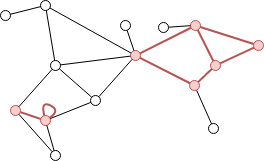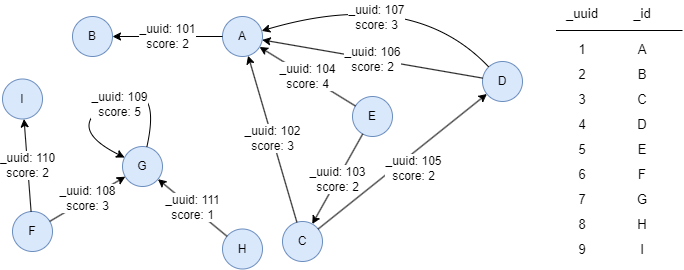# Change Nickname

Current Nickname:
Search
v4.0
v4.0

# Induced Subgraph

## Overview

Induced Subgraph algorithm can compute the corresponding induced subgraph from a given set of nodes and return the edges in that induced subgraph in the form of path. Induced subgraph, as one of the basic concepts in Graph Theory, is commonly used to dig the direct connections between target entities.

## Basic Concept

### Induced Subgraph

Specifying a set of nodes in the graph as initial nodes, and finding edges which two endpoints are both of those initial nodes, these initial nodes and edges that are found form an induced subgraph.The endpoints of all red edges in the graph above are both red nodes, while all black edges have at least one endpoint that is not red node, all red nodes and red edges in the graph form an induced subgraph.

## Special Case

### Lonely Node, Disconnected Graph

Lonely node is valid initial node.

If the given initial nodes are in different connected components of the graph, induced subgraph will be produced in each connected component.

### Self-loop Edge

Self-loop edge of the initial node must be one edge in the induced subgraph.

### Directed Edge

For directed edges, Induced Subgraph algorithm ignores the direction of edges but calculates them as undirected edges.

## Results and Statistics

Take the graph below as an example, run the Induced Subgraph algorithm:Algorithm results: Given nodes UUID = 1,3,4,7,10, return the induced subgraph (1-hop paths that form the subgraph)

1 ---- 3
3 ---- 4
4 ---- 1
4 ---- 1
7 ---- 7

Algorithm statistics: N/A

## Command and Configuration

• Command: `algo(subgraph)`
• Configurations for the parameter `params()`:
Name Type
Default
Specification
Description
ids / uuids []`_id` / []`_uuid` / / IDs or UUIDs of nodes to be calculated; all nodes to be calculated if not set
limit int -1 >=-1 Number of results to return; return all results if sets to -1 or not set

## Algorithm Execution

#### 1. File Writeback

Configuration
Data in Each Row
Description
filename `_id--[_uuid]--_id` startNode ID -- edge UUID -- endNode ID

Example: Calculate induced subgraph with nodes UUID = 1,3,4,7,10, write the algorithm results back to file named paths

``````algo(subgraph).params({
uuids: [1,3,4,7,10]
}).write({
file:{
filename: "paths"
}
})
``````

#### 2. Property Writeback

Not supported by this algorithm.

#### 3. Statistics Writeback

This algorithm has no statistics.

### Direct Return

Alias Ordinal
Type
Description
Column Name
0 []`path` 1-hop path that forms the induced subgraph, namely the startNode, edge and endNode `_uuid` --`_uuid`-- `_uuid` in one column

Example: Calculate induced subgraph with nodes UUID = 1,3,4,7,10, define algorithm results as alias named path, and return the results

``````algo(subgraph).params({
uuids: [1,3,4,7,10]
}) as path
return path
``````

### Streaming Return

Alias Ordinal
Type
Description
Column Name
0 []`path` 1-hop path that forms the induced subgraph, namely the startNode, edge and endNode `_uuid` --`_uuid`-- `_uuid` in one column

Example: Calculate induced subgraph with nodes UUID = 6,7, return the list of edge arrays

``````algo(subgraph).params({
uuids: [6,7]
}) as p
return pedges(p)
``````

### Real-time Statistics

This algorithm has no statistics.